## Example of PL/I macro

January 27, 2021 Mainframe , , ,

Up until last week PL/I macros were a bit of a mystery.  Most of the ones that I’d seen in customer code were impressively inscrutable, and if I had to look at any of them, my reaction was to throw my hands in the air and plead with the compiler backend guys for help.  Implementing one such macro has been very helpful to understanding how these work.

Here is a C program that roughly models some PL/I code of interest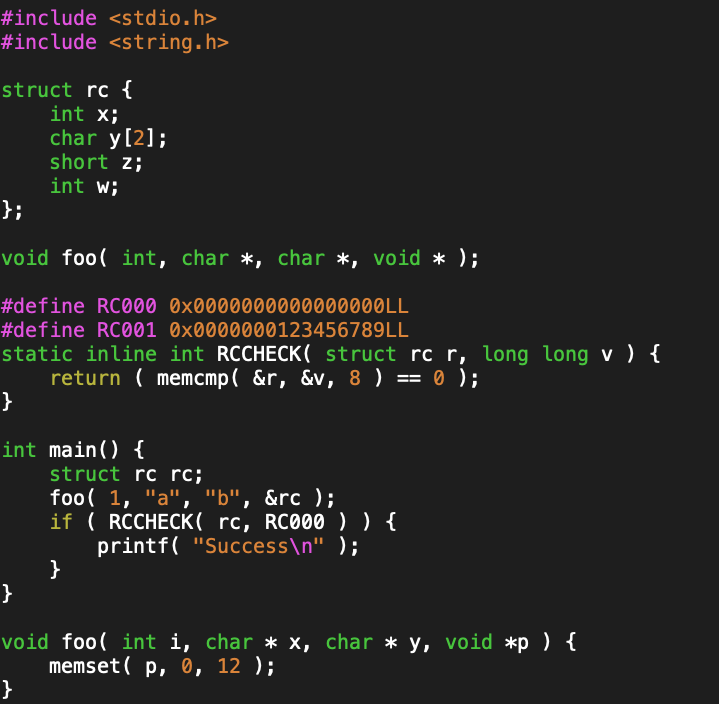The documentation for the ‘foo’ function says of the final return code parameter that it is 12 bytes long, and that the ‘rcvalues.h’ header file has a set of RCNNN constants and a RCCHECK macro that can be used to test for any one of those constants.  A possible C implementation of that header might look something like:

/* rcvalues.h */
#define RC000 0x0000000000000000LL
#define RC001 0x0000000123456789LL
/* ... */

#define RCCHECK( urc, crc ) ( memcmp( &(urc), &(crc), 8 ) == 0 )


PL/I APIs do not typically use modern constructs like typedefs.  The closest that I have seen is for an API header file (copybook in the mainframe lingo) is to declare a variable (which becomes a local variable with a specific name in the including module), which the programmer can refer to using the LIKE keyword, as in the following example: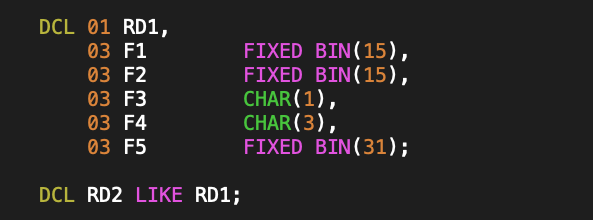I believe there is also a DEFINE keyword available in newer PL/I compilers, which provides a typedef like mechanism, but most existing code probably doesn’t use such new-fangled nonsense, when cut and paste has far superior maintenance characteristics.  For that reason, the API would be unlikely to have a typedef equivalent for the return code structure.  Instead, the PL/I equivalent of the C code above, would probably look like: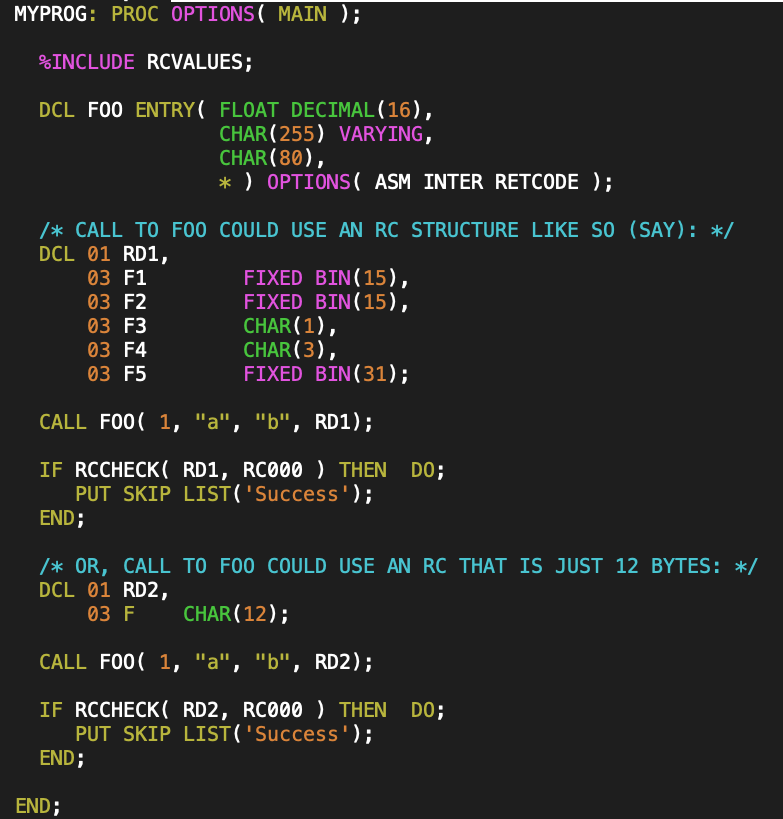(i.e. the C code is really modeled on the PL/I code of this form, and if this was a C API, the API would have a struct declaration or a typedef for the return code structure)

The RCNNN constants would actually be found as named variables (not immutable constants) in the copy book, perhaps declared something like:I struggled a bit to figure out what the PL/I equivalent of my C RCCHECK macro would be.  The following inner function correctly did the required type casting and comparisons: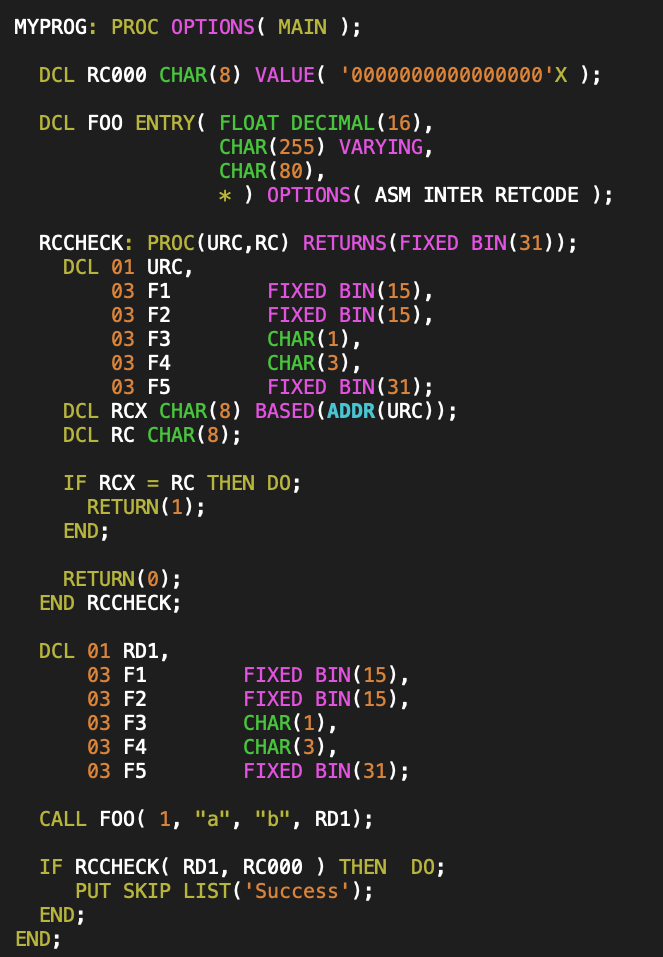The implementation is very long, since the entire declaration of the input parameter type has to be duplicated.

If I was to put this RCCHECK implementation above into my RCVALUES.inc header file, it would only work if all the customer declaration of their return code structure objects were field by field compatible.  What I really want is for my RCCHECK function to take the address of the parameter, and pass that instead of the underlying type.  That was not at all obvious to figure out how to do, but with some help, I was eventually able to construct a PL/I macro (with helper inner-function) of the following form: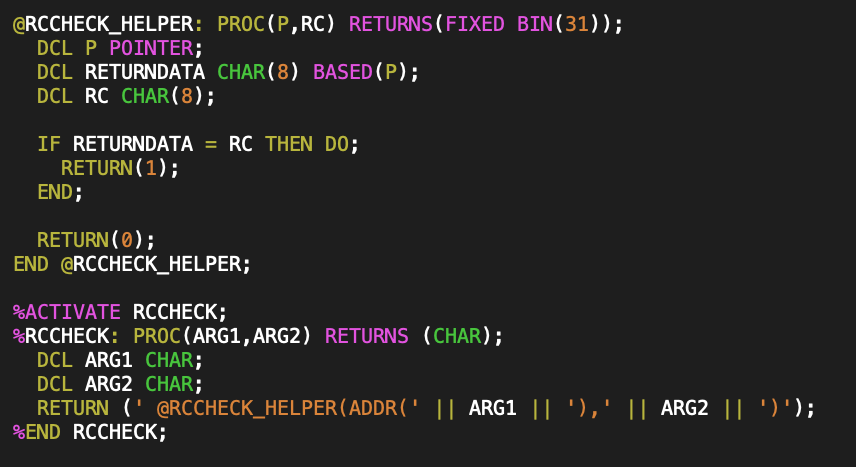It’s clearly no longer a one liner.  Some notes on this PL/I macro:

• The PL/I macro body looks like a regular PL/I function, but the begin-PROCEDURE and END statements start with % (% is not part of the PROC name.)
• Macro parameters and return values are explicit strings, regardless of the types of the parameters that were actually passed.
• In PL/I the || symbol is used for string concatenation, so this constructs output that inserts an ADDR() call around ARG1 token and then passes the ARG2 token as is.
• I don’t know if there’s a way to implement this macro in a way that doesn’t require a helper function, and still have the output work in the context of an IF statement.
• You have to explicitly enable the macro, using %ACTIVATE.  In my case, without %ACTIVATE, the RCCHECK symbol ends up as an undeclared external entry, and was no call to the @RCCHECK_HELPER function $${}^{}$$.
• Observe that the PL/I macro provides a mechanism to jam whatever you want into the code, as the compiler’s macro preprocessor replaces the macro call tokens with the string that you have provided, leaving that string for the final compiler pass to interpret instead.

If I compile the code using this macro version of RCCHECK, the preprocessor output looks like: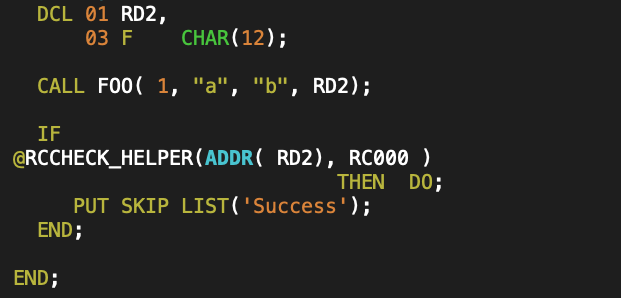I’m still pretty horrified at some of the macros that I’ve seen in customer code — they almost seem like the source equivalent of self modifying code.  You can’t figure out what is going on without also looking at all the output of the precompiler passes.  This is especially evil, since you can write PL/I preprocessor macros that generate preprocessor macros and require multiple preprocessor passes to produce the final desired output!

### Footnotes

 note that @ is a valid PL/I character to use in a symbol name, as is # and $— so if you want your functions to look like swear words, this is a language where that is possible. Something like the following is probably valid PL/I : V = #@$1A#@(1);


For added entertainment, your file names (i.e. PDS member names) can also be like ‘#@$1A#@’. Storing files with names like that on a Unix filesystem results in hours of fun, as you are then left with the task of figuring out how to properly quote file names with embedded$’s and #’s in scripts and makefiles.

## Notes.

Due to limitations in the MathJax-Latex package, all the oriented integrals in this blog post should be interpreted as having a clockwise orientation. [See the PDF version of this post for more sophisticated formatting.]

## Guts.

Given a two dimensional generating vector space, there are two instances of the fundamental theorem for multivector integration
\begin{equation}\label{eqn:unpackingFundamentalTheorem:20}
\int_S F d\Bx \lrpartial G = \evalbar{F G}{\Delta S},
\end{equation}
and
\begin{equation}\label{eqn:unpackingFundamentalTheorem:40}
\int_S F d^2\Bx \lrpartial G = \oint_{\partial S} F d\Bx G.
\end{equation}
The first case is trivial. Given a parameterizated curve $$x = x(u)$$, it just states
\begin{equation}\label{eqn:unpackingFundamentalTheorem:60}
\int_{u(0)}^{u(1)} du \PD{u}{}\lr{FG} = F(u(1))G(u(1)) – F(u(0))G(u(0)),
\end{equation}
for all multivectors $$F, G$$, regardless of the signature of the underlying space.

The surface integral is more interesting. Let’s first look at the area element for this surface integral, which is
\begin{equation}\label{eqn:unpackingFundamentalTheorem:80}
d^2 \Bx = d\Bx_u \wedge d \Bx_v.
\end{equation}
Geometrically, this has the area of the parallelogram spanned by $$d\Bx_u$$ and $$d\Bx_v$$, but weighted by the pseudoscalar of the space. This is explored algebraically in the following problem and illustrated in fig. 1.

## Problem: Expansion of 2D area bivector.

Let $$\setlr{e_1, e_2}$$ be an orthonormal basis for a two dimensional space, with reciprocal frame $$\setlr{e^1, e^2}$$. Expand the area bivector $$d^2 \Bx$$ in coordinates relating the bivector to the Jacobian and the pseudoscalar.

With parameterization $$x = x(u,v) = x^\alpha e_\alpha = x_\alpha e^\alpha$$, we have
\begin{equation}\label{eqn:unpackingFundamentalTheorem:120}
\Bx_u \wedge \Bx_v
=
\lr{ \PD{u}{x^\alpha} e_\alpha } \wedge
\lr{ \PD{v}{x^\beta} e_\beta }
=
\PD{u}{x^\alpha}
\PD{v}{x^\beta}
e_\alpha
e_\beta
=
\PD{(u,v)}{(x^1,x^2)} e_1 e_2,
\end{equation}
or
\begin{equation}\label{eqn:unpackingFundamentalTheorem:160}
\Bx_u \wedge \Bx_v
=
\lr{ \PD{u}{x_\alpha} e^\alpha } \wedge
\lr{ \PD{v}{x_\beta} e^\beta }
=
\PD{u}{x_\alpha}
\PD{v}{x_\beta}
e^\alpha
e^\beta
=
\PD{(u,v)}{(x_1,x_2)} e^1 e^2.
\end{equation}
The upper and lower index pseudoscalars are related by
\begin{equation}\label{eqn:unpackingFundamentalTheorem:180}
e^1 e^2 e_1 e_2 =
-e^1 e^2 e_2 e_1 =
-1,
\end{equation}
so with $$I = e_1 e_2$$,
\begin{equation}\label{eqn:unpackingFundamentalTheorem:200}
e^1 e^2 = -I^{-1},
\end{equation}
leaving us with
\begin{equation}\label{eqn:unpackingFundamentalTheorem:140}
d^2 \Bx
= \PD{(u,v)}{(x^1,x^2)} du dv\, I
= -\PD{(u,v)}{(x_1,x_2)} du dv\, I^{-1}.
\end{equation}
We see that the area bivector is proportional to either the upper or lower index Jacobian and to the pseudoscalar for the space.

We may write the fundamental theorem for a 2D space as
\begin{equation}\label{eqn:unpackingFundamentalTheorem:680}
\int_S du dv \, \PD{(u,v)}{(x^1,x^2)} F I \lrgrad G = \oint_{\partial S} F d\Bx G,
\end{equation}
where we have dispensed with the vector derivative and use the gradient instead, since they are identical in a two parameter two dimensional space. Of course, unless we are using $$x^1, x^2$$ as our parameterization, we still want the curvilinear representation of the gradient $$\grad = \Bx^u \PDi{u}{} + \Bx^v \PDi{v}{}$$.

## Problem: Standard basis expansion of fundamental surface relation.

For a parameterization $$x = x^1 e_1 + x^2 e_2$$, where $$\setlr{ e_1, e_2 }$$ is a standard (orthogonal) basis, expand the fundamental theorem for surface integrals for the single sided $$F = 1$$ case. Consider functions $$G$$ of each grade (scalar, vector, bivector.)

From \ref{eqn:unpackingFundamentalTheorem:140} we see that the fundamental theorem takes the form
\begin{equation}\label{eqn:unpackingFundamentalTheorem:220}
\int_S dx^1 dx^2\, F I \lrgrad G = \oint_{\partial S} F d\Bx G.
\end{equation}
In a Euclidean space, the operator $$I \lrgrad$$, is a $$\pi/2$$ rotation of the gradient, but has a rotated like structure in all metrics:
\begin{equation}\label{eqn:unpackingFundamentalTheorem:240}
=
e_1 e_2 \lr{ e^1 \partial_1 + e^2 \partial_2 }
=
-e_2 \partial_1 + e_1 \partial_2.
\end{equation}

• $$F = 1$$ and $$G \in \bigwedge^0$$ or $$G \in \bigwedge^2$$. For $$F = 1$$ and scalar or bivector $$G$$ we have
\begin{equation}\label{eqn:unpackingFundamentalTheorem:260}
\int_S dx^1 dx^2\, \lr{ -e_2 \partial_1 + e_1 \partial_2 } G = \oint_{\partial S} d\Bx G,
\end{equation}
where, for $$x^1 \in [x^1(0),x^1(1)]$$ and $$x^2 \in [x^2(0),x^2(1)]$$, the RHS written explicitly is
\begin{equation}\label{eqn:unpackingFundamentalTheorem:280}
\oint_{\partial S} d\Bx G
=
\int dx^1 e_1
\lr{ G(x^1, x^2(1)) – G(x^1, x^2(0)) }
– dx^2 e_2
\lr{ G(x^1(1),x^2) – G(x^1(0), x^2) }.
\end{equation}
This is sketched in fig. 2. Since a 2D bivector $$G$$ can be written as $$G = I g$$, where $$g$$ is a scalar, we may write the pseudoscalar case as
\begin{equation}\label{eqn:unpackingFundamentalTheorem:300}
\int_S dx^1 dx^2\, \lr{ -e_2 \partial_1 + e_1 \partial_2 } g = \oint_{\partial S} d\Bx g,
\end{equation}
after right multiplying both sides with $$I^{-1}$$. Algebraically the scalar and pseudoscalar cases can be thought of as identical scalar relationships.
• $$F = 1, G \in \bigwedge^1$$. For $$F = 1$$ and vector $$G$$ the 2D fundamental theorem for surfaces can be split into scalar
\begin{equation}\label{eqn:unpackingFundamentalTheorem:320}
\int_S dx^1 dx^2\, \lr{ -e_2 \partial_1 + e_1 \partial_2 } \cdot G = \oint_{\partial S} d\Bx \cdot G,
\end{equation}
and bivector relations
\begin{equation}\label{eqn:unpackingFundamentalTheorem:340}
\int_S dx^1 dx^2\, \lr{ -e_2 \partial_1 + e_1 \partial_2 } \wedge G = \oint_{\partial S} d\Bx \wedge G.
\end{equation}
To expand \ref{eqn:unpackingFundamentalTheorem:320}, let
\begin{equation}\label{eqn:unpackingFundamentalTheorem:360}
G = g_1 e^1 + g_2 e^2,
\end{equation}
for which
\begin{equation}\label{eqn:unpackingFundamentalTheorem:380}
\lr{ -e_2 \partial_1 + e_1 \partial_2 } \cdot G
=
\lr{ -e_2 \partial_1 + e_1 \partial_2 } \cdot
\lr{ g_1 e^1 + g_2 e^2 }
=
\partial_2 g_1 – \partial_1 g_2,
\end{equation}
and
\begin{equation}\label{eqn:unpackingFundamentalTheorem:400}
d\Bx \cdot G
=
\lr{ dx^1 e_1 – dx^2 e_2 } \cdot \lr{ g_1 e^1 + g_2 e^2 }
=
dx^1 g_1 – dx^2 g_2,
\end{equation}
so \ref{eqn:unpackingFundamentalTheorem:320} expands to
\begin{equation}\label{eqn:unpackingFundamentalTheorem:500}
\int_S dx^1 dx^2\, \lr{ \partial_2 g_1 – \partial_1 g_2 }
=
\int
\evalbar{dx^1 g_1}{\Delta x^2} – \evalbar{ dx^2 g_2 }{\Delta x^1}.
\end{equation}
This coordinate expansion illustrates how the pseudoscalar nature of the area element results in a duality transformation, as we end up with a curl like operation on the LHS, despite the dot product nature of the decomposition that we used. That can also be seen directly for vector $$G$$, since
\begin{equation}\label{eqn:unpackingFundamentalTheorem:560}
=
=
dA I \lr{ \grad \wedge G },
\end{equation}
since the scalar selection of $$I \lr{ \grad \cdot G }$$ is zero.In the grade-2 relation \ref{eqn:unpackingFundamentalTheorem:340}, we expect a pseudoscalar cancellation on both sides, leaving a scalar (divergence-like) relationship. This time, we use upper index coordinates for the vector $$G$$, letting
\begin{equation}\label{eqn:unpackingFundamentalTheorem:440}
G = g^1 e_1 + g^2 e_2,
\end{equation}
so
\begin{equation}\label{eqn:unpackingFundamentalTheorem:460}
\lr{ -e_2 \partial_1 + e_1 \partial_2 } \wedge G
=
\lr{ -e_2 \partial_1 + e_1 \partial_2 } \wedge G
\lr{ g^1 e_1 + g^2 e_2 }
=
e_1 e_2 \lr{ \partial_1 g^1 + \partial_2 g^2 },
\end{equation}
and
\begin{equation}\label{eqn:unpackingFundamentalTheorem:480}
d\Bx \wedge G
=
\lr{ dx^1 e_1 – dx^2 e_2 } \wedge
\lr{ g^1 e_1 + g^2 e_2 }
=
e_1 e_2 \lr{ dx^1 g^2 + dx^2 g^1 }.
\end{equation}
So \ref{eqn:unpackingFundamentalTheorem:340}, after multiplication of both sides by $$I^{-1}$$, is
\begin{equation}\label{eqn:unpackingFundamentalTheorem:520}
\int_S dx^1 dx^2\,
\lr{ \partial_1 g^1 + \partial_2 g^2 }
=
\int
\evalbar{dx^1 g^2}{\Delta x^2} + \evalbar{dx^2 g^1 }{\Delta x^1}.
\end{equation}

As before, we’ve implicitly performed a duality transformation, and end up with a divergence operation. That can be seen directly without coordinate expansion, by rewriting the wedge as a grade two selection, and expanding the gradient action on the vector $$G$$, as follows
\begin{equation}\label{eqn:unpackingFundamentalTheorem:580}
=
=
dA I \lr{ \grad \cdot G },
\end{equation}
since $$I \lr{ \grad \wedge G }$$ has only a scalar component.

## Theorem 1.1: Green’s theorem .

Let $$S$$ be a Jordan region with a piecewise-smooth boundary $$C$$. If $$P, Q$$ are continuously differentiable on an open set that contains $$S$$, then
\begin{equation*}
\int dx dy \lr{ \PD{y}{P} – \PD{x}{Q} } = \oint P dx + Q dy.
\end{equation*}

## Problem: Relationship to Green’s theorem.

If the space is Euclidean, show that \ref{eqn:unpackingFundamentalTheorem:500} and \ref{eqn:unpackingFundamentalTheorem:520} are both instances of Green’s theorem with suitable choices of $$P$$ and $$Q$$.

I will omit the subtleties related to general regions and consider just the case of an infinitesimal square region.

### Start proof:

Let’s start with \ref{eqn:unpackingFundamentalTheorem:500}, with $$g_1 = P$$ and $$g_2 = Q$$, and $$x^1 = x, x^2 = y$$, the RHS is
\begin{equation}\label{eqn:unpackingFundamentalTheorem:600}
\int dx dy \lr{ \PD{y}{P} – \PD{x}{Q} }.
\end{equation}
On the RHS we have
\begin{equation}\label{eqn:unpackingFundamentalTheorem:620}
\int \evalbar{dx P}{\Delta y} – \evalbar{ dy Q }{\Delta x}
=
\int dx \lr{ P(x, y_1) – P(x, y_0) } – \int dy \lr{ Q(x_1, y) – Q(x_0, y) }.
\end{equation}
This pair of integrals is plotted in fig. 3, from which we see that \ref{eqn:unpackingFundamentalTheorem:620} can be expressed as the line integral, leaving us with
\begin{equation}\label{eqn:unpackingFundamentalTheorem:640}
\int dx dy \lr{ \PD{y}{P} – \PD{x}{Q} }
=
\oint dx P + dy Q,
\end{equation}
which is Green’s theorem over the infinitesimal square integration region.

For the equivalence of \ref{eqn:unpackingFundamentalTheorem:520} to Green’s theorem, let $$g^2 = P$$, and $$g^1 = -Q$$. Plugging into the LHS, we find the Green’s theorem integrand. On the RHS, the integrand expands to
\begin{equation}\label{eqn:unpackingFundamentalTheorem:660}
\evalbar{dx g^2}{\Delta y} + \evalbar{dy g^1 }{\Delta x}
=
dx \lr{ P(x,y_1) – P(x, y_0)}
+
dy \lr{ -Q(x_1, y) + Q(x_0, y)},
\end{equation}
which is exactly what we found in \ref{eqn:unpackingFundamentalTheorem:620}.

### End proof.

We may also relate multivector gradient integrals in 2D to the normal integral around the boundary of the bounding curve. That relationship is as follows.

## Theorem 1.2: 2D gradient integrals.

\begin{equation*}
\begin{aligned}
\int J du dv \rgrad G &= \oint I^{-1} d\Bx G = \int J \lr{ \Bx^v du + \Bx^u dv } G \\
\int J du dv F \lgrad &= \oint F I^{-1} d\Bx = \int J F \lr{ \Bx^v du + \Bx^u dv },
\end{aligned}
\end{equation*}
where $$J = \partial(x^1, x^2)/\partial(u,v)$$ is the Jacobian of the parameterization $$x = x(u,v)$$. In terms of the coordinates $$x^1, x^2$$, this reduces to
\begin{equation*}
\begin{aligned}
\int dx^1 dx^2 \rgrad G &= \oint I^{-1} d\Bx G = \int \lr{ e^2 dx^1 + e^1 dx^2 } G \\
\int dx^1 dx^2 F \lgrad &= \oint G I^{-1} d\Bx = \int F \lr{ e^2 dx^1 + e^1 dx^2 }.
\end{aligned}
\end{equation*}
The vector $$I^{-1} d\Bx$$ is orthogonal to the tangent vector along the boundary, and for Euclidean spaces it can be identified as the outwards normal.

### Start proof:

Respectively setting $$F = 1$$, and $$G = 1$$ in \ref{eqn:unpackingFundamentalTheorem:680}, we have
\begin{equation}\label{eqn:unpackingFundamentalTheorem:940}
\int I^{-1} d^2 \Bx \rgrad G = \oint I^{-1} d\Bx G,
\end{equation}
and
\begin{equation}\label{eqn:unpackingFundamentalTheorem:960}
\int F d^2 \Bx \lgrad I^{-1} = \oint F d\Bx I^{-1}.
\end{equation}
Starting with \ref{eqn:unpackingFundamentalTheorem:940} we find
\begin{equation}\label{eqn:unpackingFundamentalTheorem:700}
\int I^{-1} J du dv I \rgrad G = \oint d\Bx G,
\end{equation}
to find $$\int dx^1 dx^2 \rgrad G = \oint I^{-1} d\Bx G$$, as desireed. In terms of a parameterization $$x = x(u,v)$$, the pseudoscalar for the space is
\begin{equation}\label{eqn:unpackingFundamentalTheorem:720}
I = \frac{\Bx_u \wedge \Bx_v}{J},
\end{equation}
so
\begin{equation}\label{eqn:unpackingFundamentalTheorem:740}
I^{-1} = \frac{J}{\Bx_u \wedge \Bx_v}.
\end{equation}
Also note that $$\lr{\Bx_u \wedge \Bx_v}^{-1} = \Bx^v \wedge \Bx^u$$, so
\begin{equation}\label{eqn:unpackingFundamentalTheorem:760}
I^{-1} = J \lr{ \Bx^v \wedge \Bx^u },
\end{equation}
and
\begin{equation}\label{eqn:unpackingFundamentalTheorem:780}
I^{-1} d\Bx
= I^{-1} \cdot d\Bx
= J \lr{ \Bx^v \wedge \Bx^u } \cdot \lr{ \Bx_u du – \Bx_v dv }
= J \lr{ \Bx^v du + \Bx^u dv },
\end{equation}
so the right acting gradient integral is
\begin{equation}\label{eqn:unpackingFundamentalTheorem:800}
\int J du dv \grad G =
\int
\evalbar{J \Bx^v G}{\Delta v} du + \evalbar{J \Bx^u G dv}{\Delta u},
\end{equation}
which we write in abbreviated form as $$\int J \lr{ \Bx^v du + \Bx^u dv} G$$.

For the $$G = 1$$ case, from \ref{eqn:unpackingFundamentalTheorem:960} we find
\begin{equation}\label{eqn:unpackingFundamentalTheorem:820}
\int J du dv F I \lgrad I^{-1} = \oint F d\Bx I^{-1}.
\end{equation}
However, in a 2D space, regardless of metric, we have $$I a = – a I$$ for any vector $$a$$ (i.e. $$\grad$$ or $$d\Bx$$), so we may commute the outer pseudoscalars in
\begin{equation}\label{eqn:unpackingFundamentalTheorem:840}
\int J du dv F I \lgrad I^{-1} = \oint F d\Bx I^{-1},
\end{equation}
so
\begin{equation}\label{eqn:unpackingFundamentalTheorem:850}
-\int J du dv F I I^{-1} \lgrad = -\oint F I^{-1} d\Bx.
\end{equation}
After cancelling the negative sign on both sides, we have the claimed result.

To see that $$I a$$, for any vector $$a$$ is normal to $$a$$, we can compute the dot product
\begin{equation}\label{eqn:unpackingFundamentalTheorem:860}
\lr{ I a } \cdot a
=
=
= 0,
\end{equation}
since the scalar selection of a bivector is zero. Since $$I^{-1} = \pm I$$, the same argument shows that $$I^{-1} d\Bx$$ must be orthogonal to $$d\Bx$$.

### End proof.

Let’s look at the geometry of the normal $$I^{-1} \Bx$$ in a couple 2D vector spaces. We use an integration volume of a unit square to simplify the boundary term expressions.

• Euclidean: With a parameterization $$x(u,v) = u\Be_1 + v \Be_2$$, and Euclidean basis vectors $$(\Be_1)^2 = (\Be_2)^2 = 1$$, the fundamental theorem integrated over the rectangle $$[x_0,x_1] \times [y_0,y_1]$$ is
\begin{equation}\label{eqn:unpackingFundamentalTheorem:880}
\int dx dy \grad G =
\int
\Be_2 \lr{ G(x,y_1) – G(x,y_0) } dx +
\Be_1 \lr{ G(x_1,y) – G(x_0,y) } dy,
\end{equation}
Each of the terms in the integrand above are illustrated in fig. 4, and we see that this is a path integral weighted by the outwards normal.

• Spacetime: Let $$x(u,v) = u \gamma_0 + v \gamma_1$$, where $$(\gamma_0)^2 = -(\gamma_1)^2 = 1$$. With $$u = t, v = x$$, the gradient integral over a $$[t_0,t_1] \times [x_0,x_1]$$ of spacetime is
\begin{equation}\label{eqn:unpackingFundamentalTheorem:900}
\begin{aligned}
&=
\int
\gamma^1 dt \lr{ G(t, x_1) – G(t, x_0) }
+
\gamma^0 dx \lr{ G(t_1, x) – G(t_1, x) } \\
&=
\int
\gamma_1 dt \lr{ -G(t, x_1) + G(t, x_0) }
+
\gamma_0 dx \lr{ G(t_1, x) – G(t_1, x) }
.
\end{aligned}
\end{equation}
With $$t$$ plotted along the horizontal axis, and $$x$$ along the vertical, each of the terms of this integrand is illustrated graphically in fig. 5. For this mixed signature space, there is no longer any good geometrical characterization of the normal.

• Spacelike:
Let $$x(u,v) = u \gamma_1 + v \gamma_2$$, where $$(\gamma_1)^2 = (\gamma_2)^2 = -1$$. With $$u = x, v = y$$, the gradient integral over a $$[x_0,x_1] \times [y_0,y_1]$$ of this space is
\begin{equation}\label{eqn:unpackingFundamentalTheorem:920}
\begin{aligned}
&=
\int
\gamma^2 dx \lr{ G(x, y_1) – G(x, y_0) }
+
\gamma^1 dy \lr{ G(x_1, y) – G(x_1, y) } \\
&=
\int
\gamma_2 dx \lr{ -G(x, y_1) + G(x, y_0) }
+
\gamma_1 dy \lr{ -G(x_1, y) + G(x_1, y) }
.
\end{aligned}
\end{equation}
Referring to fig. 6. where the elements of the integrand are illustrated, we see that the normal $$I^{-1} d\Bx$$ for the boundary of this region can be characterized as inwards.

# References

 S.L. Salas and E. Hille. Calculus: one and several variables. Wiley New York, 1990.

## Switching from screen to tmux

January 11, 2021 perl and general scripting hackery , ,

RHEL8 (Redhat enterprise Linux 8) has dropped support for my old friend screen.  I had found a package somewhere that still worked for one new RHEL8 installation, but didn’t record where, and the version I installed on my most recently upgraded machine is crashing horribly.

### Screen

Screen was originally recommended to me by Sam Bortman when I worked at IBM, and I am forever grateful to him, as it has been a godsend over the years.  The basic idea is that you have have a single terminal session that not only saves all state, but also allows you to have multiple terminal “tabs” all controlled by that single master session.  Since then, I no longer use nohup, and no longer try to run many background jobs anymore.  Both attempting to background or nohup a job can be problematic, as there are a suprising number of tools and scripts that expect an active terminal.  As well as the multiplexing functionality, running screen ensures that if you loose your network connection, or switch from wired to wireless and back, or go home or go to work, in all cases, you can resume your work where you left it.

A typical session looks something like the following:

i.e. plain old terminal, but three little “tabs” at the bottom, each representing a different shell on the same machine.  In this case, I have my ovpn client running in window 0, am in my Tests/scripts/ directory in window 1, and have ‘git log –graph –decorate’ running in window 2.  The second screenshot above shows the screen menu, listing all the different active windows.

screen can do window splitting vertically and horizontally too, but I’ve never used that.  My needs are pretty simple:

• multiple windows, each with a different shell,
• an easy way to tab between the windows,
• an easy way to start a new shell.

I always found the screen key bindings to be somewhat cumbersome (example: control-A ” to start the window menu), and it didn’t take me long before I’d constructed a standard .screenrc for myself with a couple handy key bindings:

• F5: -1th window
• F6: previous window (after switching explicitly using key bindings or the menu)
• F8: +1th window
• F9: new window

I’ve used those key bindings for so many years that I feel lost without them!

With screen crashing on my constantly, my options were to find a stable package somewhere, build it myself (which I used to do all the time at IBM when I had to work on many Unix platforms simultaneously), or bite the bullet and see what it would take to switch to tmux.

### tmux attach

I chose the latter, and with the help of some tutorials, it was pretty easy to make the switch to tmux.  Startup is pretty easy:

tmux


and

tmux at


(at is short for attach, what to use instead of screen -dr)

### tmux bindings

All my trusty key bindings were easy to reimplement, requiring the following in my .tmux.conf:

bind-key -T root F4 list-windows
bind-key -T root F5 select-window -p
bind-key -T root F6 select-window -l
bind-key -T root F8 select-window -n
bind-key -T root F9 new-window


### tmux command line

One of the nice things about tmux is that you don’t need a whole bunch of complex key bindings that are hard to remember, as you can do it all on the command line from within any tmux slave window. This means that you can also alias your tmux commands easily! Here are a couple examples:

alias weekly='tmux new-window -c ~/weeklyreports/01 -n weekly -t 1'
alias master='tmux new-window -n master -c ~/master'
alias tests='tmux new-window -n tests -c ~/Tests'


These new-window aliases change the name displayed in the bottom bar, and open a new terminal in a set of specific directories.

The UI is pretty much identical, and a session might look something like: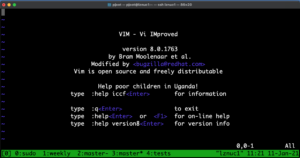### tmux prefix binding

The only other customization that I made to tmux was to override the default key binding, as tmux uses control-b instead of screen’s control-a. control-b is much easier to type than control-a, but messes up paging in vim, so I’ve reset it to control-n using:

unbind C-b
set -g prefix ^N
bind n send-prefix


With this, the rename window command becomes ‘control-n ,’.

I can’t think of anything that uses control-n, but if that choice ends up being intrusive, I’ll probably just unbind control-b and not bother with a prefix binding, since tmux has the full functioning command line options, and I can use easier to remember (or lookup) aliases.

### Incompatibilities.

It looks like the bindings that I used above are valid with RHEL8 tmux-2.7, but not with RHEL7’s tmux-1.8.  That’s a bit of a pain, and means that I’ll have to

1. find alternate newer tmux packages for RHEL7, or
2. figure out how to do the same bindings with tmux-1.8 and have different dot files, or
3. keep on using screen until I’ve managed to upgrade all my machines to RHEL8.

Nothing is ever easy;)

## Raccoons vs. Cake: “Oh, come on kids, …, it’s still good!”

January 10, 2021 Incoherent ramblings ,

Life comes in cycles, and here’s an old chapter replaying itself.

When I was a teenager, we spent weekdays with mom, and weekends with dad. Both of them lived a subsistence existence, but with the rent expenses that mom also had, she really struggled to pay the bills at that stage of our lives. I don’t remember the occasion, but one hot summer day, she had saved enough to buy the eggs, flour and other ingredients that she needed to make us all a cake as a special treat. After the cake was cooked, she put it on the kitchen table to cool enough that she could ice it (she probably would have used her classic cream-cheese and sugar recipe.)

That rental property did not have air conditioning, and the doors were always wide open in the summer. Imagine the smell of fresh baked cake pervading the air in the house, and then a blood curdling scream. It was the scream of a horrific physical injury, perhaps that of somebody with a foreign object embedding deep in the flesh of their leg. We all rushed down to find out what happened, and it turned out that the smell of the cake was not just inviting to us, but also to a family of raccoons. Mom walked into the kitchen to find a mother raccoon and her little kids all sitting politely at the table in a circle around the now cool cake, helping themselves to dainty little handfuls.  What sounded like the scream of mortal injury, was the scream of a struggling mom, who’s plan to spoil her kids was being eaten in front of her eyes.

From the kitchen you could enter the back room, or the hallway to the front door, and from the front door you could enter the “piano room”, which also had a door to the back room and back to the kitchen.  The scene degenerates into chaos at this point, with mom and the rest of us chasing crying and squealing raccoons in circles all around the first floor of the house along that circular path, with cake crumbs flying in all directions.  I don’t know how many laps we and the raccoons made of the house before we managed to shoo them all out the front or back door, but eventually we were left with just the crumb trail and the remains of the cake.

The icing on the cake was mom’s reaction though. She went over to the cake and cut all the raccoon handprints out of it. We didn’t want to eat it, and I still remember her pleading with us, “Oh come on kids, try it. It’s still good!” Poor mom.  She even took sample bites from the cake to demonstrate it was still edible, and convince us to partake in the treat that she’d worked so hard to make for us.  I don’t think that we ate her cake, despite her pleading.

Thirty years later, it’s my turn. I spent an hour making chili today, and after dinner I put the left overs out on the back porch to cool in the slow cooker pot with the lid on. I’d planned to bag and freeze part of it, and put the rest in the fridge as leftovers for the week. It was cold enough out that I didn’t think that the raccoons would be out that early, but figured it would have been fair game had I left it out all night in the “outside fridge”. Well, those little buggers were a lot more industrious than I gave them credit, and by the time I’d come back from walking the dog, they’d helped themselves to a portion, lifting the lid of the slow cooker pot, and making a big mess of as much chili as they wanted.  They ate quite a lot, but perhaps it had more spice than they cared for, as they left quite a lot:

Judging by the chili covered hand prints on the back deck I think they enjoyed themselves, despite the spices.

When I went upstairs to let Sofia know what had happened, she immediately connected the dots to this cake story that I’ve told so many times, and said in response: “Oh come on kids, it’s still good!”, at which point we both started laughing.

The total cost of the chili itself was probably only \$17, plus one hour of time.  However, I didn’t intend to try to talk anybody into eating the remains.  It is just not worth getting raccoon carried Giardia or some stomach bug.  I was sad to see my work wasted and the leftovers ruined.  I wish Mom was still with us, so that I could share this with her.  I can imagine her visiting on this very day, where I could have scooped everything off the top, and then offered her a spoonful, saying “Oh come on Mom, it’s still good!”  I think that she would have gotten a kick out of that, even if she was always embarrassed about this story and how poor we were at the time.

### Final thoughts.

There were 4 cans of beans in that pot of chili.  I have to wonder if we are going to have a family of farting raccoons in the neighbourhood for a few days?

## Some experiments in youtube mathematics videos

A couple years ago I was curious how easy it would be to use a graphics tablet as a virtual chalkboard, and produced a handful of very rough YouTube videos to get a feel for the basics of streaming and video editing (much of which I’ve now forgotten how to do). These were the videos in chronological order:

• Introduction to Geometric (Clifford) Algebra.Introduction to Geometric (Clifford) algebra. Interpretation of products of unit vectors, rules for reducing products of unit vectors, and the axioms that justify those rules.
• Geometric Algebra: dot, wedge, cross and vector products.Geometric (Clifford) Algebra introduction, showing the relation between the vector product dot and wedge products, and the cross product.
• Solution of two line intersection using geometric algebra.
• Linear system solution using the wedge product.. This video provides a standalone introduction to the wedge product, the geometry of the wedge product and some properties, and linear system solution as a sample application. In this video the wedge product is introduced independently of any geometric (Clifford) algebra, as an antisymmetric and associative operator. You’ll see that we get Cramer’s rule for free from this solution technique.
• Exponential form of vector products in geometric algebra.In this video, I discussed the exponential form of the product of two vectors.

I showed an example of how two unit vectors, each rotations of zcap orthonormal $$\mathbb{R}^3$$ planes, produce a “complex” exponential in the plane that spans these two vectors.

• Velocity and acceleration in cylindrical coordinates using geometric algebra.I derived the cylindrical coordinate representations of the velocity and acceleration vectors, showing the radial and azimuthal components of each vector.

I also showed how these are related to the dot and wedge product with the radial unit vector.

• Duality transformations in geometric algebra.Duality transformations (pseudoscalar multiplication) will be demonstrated in $$\mathbb{R}^2$$ and $$\mathbb{R}^3$$.

A polar parameterized vector in $$\mathbb{R}^2$$, written in complex exponential form, is multiplied by a unit pseudoscalar for the x-y plane. We see that the result is a vector normal to that vector, with the direction of the normal dependent on the order of multiplication, and the orientation of the pseudoscalar used.

In $$\mathbb{R}^3$$ we see that a vector multiplied by a pseudoscalar yields the bivector that represents the plane that is normal to that vector. The sign of that bivector (or its cyclic orientation) depends on the orientation of the pseudoscalar. The order of multiplication was not mentioned in this case since the $$\mathbb{R}^3$$ pseudoscalar commutes with any grade object (assumed, not proved). An example of a vector with two components in a plane, multiplied by a pseudoscalar was also given, which allowed for a visualization of the bivector that is normal to the original vector.

• Math bait and switch: Fractional integer exponents.When I was a kid, my dad asked me to explain fractional exponents, and perhaps any non-positive integer exponents, to him. He objected to the idea of multiplying something by itself $$1/2$$ times.

I failed to answer the question to his satisfaction. My own son is now reviewing the rules of exponentiation, and it occurred to me (30 years later) why my explanation to Dad failed.

Essentially, there’s a small bait and switch required, and my dad didn’t fall for it.

The meaning that my dad gave to exponentiation was that $$x^n$$ equals $$x$$ times itself $$n$$ times.

Using this rule, it is easy to demonstrate that $$x^a x^b = x^{a + b}$$, and this can be used to justify expressions like $$x^{1/2}$$. However, doing this really means that we’ve switched the definition of exponential, defining an exponential as any number that satisfies the relationship:

$$x^a x^b = x^{a+b}$$,

where $$x^1 = x$$. This slight of hand is required to give meaning to $$x^{1/2}$$ or other exponentials where the exponential argument is any non-positive integer.

Of these videos I just relistened to the wedge product episode, as I had a new lone comment on it, and I couldn’t even remember what I had said. It wasn’t completely horrible, despite the low tech. I was, however, very surprised how soft and gentle my voice was. When I am talking math in person, I get very animated, but attempting to manage the tech was distracting and all the excitement that I’d normally have was obliterated.

I’d love to attempt a manim based presentation of some of this material, but suspect if I do something completely scripted like that, I may not be a very good narrator.